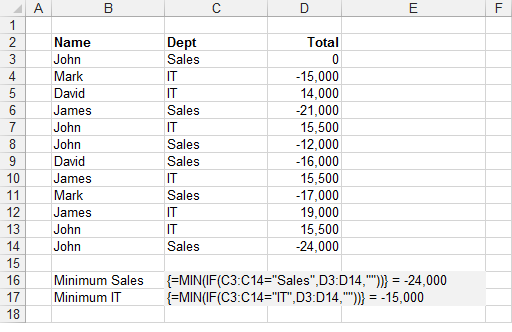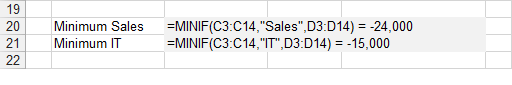### MINIF

Returns the minimum value in a range of values that match a certain condition.

`'rgeCriteria - The range of cells containing the criteria you want to check.'sCriteria - The criteria value you want to match.'rgeMinRange - The range of corresponding values you want the minimum of.Public Function MINIF(ByVal rgeCriteria As Range, _                       ByVal sCriteria As String, _                       ByVal rgeMinRange As Range) As Single           Dim iconditioncolno As Integer Dim inumberscolno As Integer Dim lrowno As Long Dim sngmin As Single Dim vcellvalue As Variant    iconditioncolno = rgeCriteria.Column    inumberscolno = rgeMinRange.Column    For lrowno = 1 To rgeCriteria.Rows.Count       vcellvalue = rgeCriteria.Parent.Cells(rgeCriteria.Row + lrowno - 1, inumberscolno).Value       If rgeCriteria.Parent.Cells(rgeCriteria.Row + lrowno - 1, iconditioncolno).Value = sCriteria And _          IsNumeric(vcellvalue) = True Then          If sngmin = 0 Then sngmin = vcellvalue          If vcellvalue < sngmin Then sngmin = vcellvalue       End If             If sngmin <> 0 And IsEmpty(vcellvalue) = True Then Exit For    Next lrowno        For lrowno = 1 To rgeCriteria.Rows.Count       vcellvalue = rgeCriteria.Parent.Cells(rgeCriteria.Row + lrowno - 1, inumberscolno).Value       If rgeCriteria.Parent.Cells(rgeCriteria.Row + lrowno - 1, iconditioncolno).Value = sCriteria And _          IsNumeric(vcellvalue) = True Then          If rgeCriteria.Parent.Cells(rgeCriteria.Row + lrowno - 1, inumberscolno).Value < sngmin Then             sngmin = rgeCriteria.Parent.Cells(rgeCriteria.Row + lrowno - 1, inumberscolno).Value          End If       End If             If sngmin <> 0 And IsEmpty(vcellvalue) = True Then Exit For    Next lrowno        MINIF = sngmin End Function `

MINIFS was added as a built-in function in 2016 Jan.
There is an Array Formula equivalent if you do not want to use the user defined function.
This includes hidden rows.
This user defined function is similar to the built-in SUMIF function.#### Array Formula

To find out more about Array Formulas please refer to the Array Formulas section.
To find out more about the individual functions please refer to the MIN function and IF function pages.
This example illustrates how to use an array formula to obtain the minimum value in a column based on a condition.The formula in row 16 returns the smallest total in the "Sales" department.
The formula in row 17 returns the smallest total in the "IT" department.
The formula [C3:C14="Sales"] returns an array of 1's and 0's indicating if the value in column "C" satisfies the condition.
Every number 1 in this array represents the value True in the IF statement and therefore has its corresponding value returned from column "D".
Every number 0 in this array represents the value False in the IF statement and therefore an empty string is returned in this case. The MIN function will ignore any string values.
The use of the empty string is to ensure that the formula works with both positive and negative numbers.
This array of numbers is then passed to the MIN() function to obtain the largest number.
Remember that you must press (Ctrl + Shift + Enter) to enter the formulas.

#### Important

You cannot use column references (such as "A:A" or "D:D") in your array formulas.
Any cell ranges passed to an array formula must have the same number of rows. This is to ensure that all the temporary arrays used are the same length.